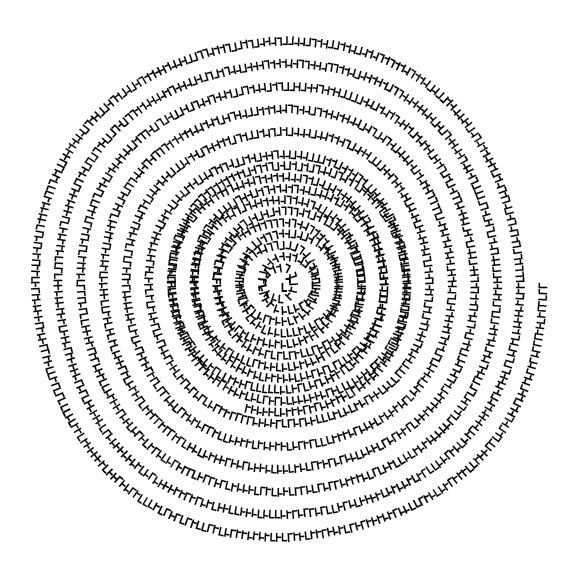# Spiro Text

extendscript, typography, spiro-text, repeat, unicode, chance

This example adds text to a path in InDesign. It takes random characters from an array of unicode signs and adds them to the path.``````var doc = app.activeDocument;
var pw = doc.documentPreferences.pageWidth;
var ph = doc.documentPreferences.pageHeight;
// the page is already there
var page = doc.pages.item(0);
page.pageItems.everyItem().remove(); // clear the page
// get the center of the page
var cx = pw / 2;
var cy = ph / 2;
// define the initial radius
var init_rad = 0.9 * (pw / 2);
// create a graphicLine
var gl = page.graphicLines.add();
var radians_angle; // this will hold the angle in radians
var rounds = 20; // how many rounds
//   we need to count the path points.
//   the first an second always exist in  a graphic line
var count = 0;
var degree_step = 4;
// loop through the data.anchors
for (var degrees = 0; degrees < 360 * rounds; degrees += (360 / degree_step)) {
// calculate the degree to radians
radians_angle = ((degrees / 360) % 360) * 2 * Math.PI;
// get the point
var point = gl.paths.pathPoints[count];
// set the disturb to 0 if you want straight lines
// calc the position on the circle
var x1 = cx + (radius) * Math.cos(radians_angle);
var y1 = cy + (radius) * Math.sin(radians_angle);
/**
* a graphicLine always has 2 path points
* so we need to add points only from the third index
*
*/
if (count < 2) {
point.anchor = [x1, y1];
if (count % 4 === 0) {
point.rightDirection = [x1, y1 + radius];
}
if (count % 4 === 1) {
point.rightDirection = [x1 - radius, y1];
}
if (count % 4 === 2) {
point.rightDirection = [x1, y1 - radius];
}
if (count % 4 === 3) {
point.rightDirection = [x1 + radius, y1];
}
} else {
point.anchor = [x1, y1];
if (count % 4 === 0) {
point.rightDirection = [x1, y1 + radius];
}
if (count % 4 === 1) {
point.rightDirection = [x1 - radius, y1];
}
if (count % 4 === 2) {
point.rightDirection = [x1, y1 - radius];
}
if (count % 4 === 3) {
point.rightDirection = [x1 + radius, y1];
}
}
// now make the circle smaller by every round
count++;
}
// now for the textPath
var tp = gl.textPaths.add(); // add a textPath to the line
// we do some JS magic.
// This is a inline function that gets executed right away
// and returns a set of unicode characters
var text = (function() {
var arr = ['\u02e5', '\u02e6', '\u02e7', '\u02e8', '\u02e9'];
var str = '';
for (var i = 0; i < 2000; i++) {
str += arr[Math.floor(Math.random() * arr.length)];
}
return str;
})(this);
tp.contents = text; // add the text to the textPath
// and try to set the font.
// Alert the user if it does not work
try {
tp.paragraphs.everyItem().appliedFont = app.fonts.item('Helvetica\tBold');
} catch (e) {
alert('This script needs Helvetica Bold');
}
var path = File(\$.fileName).parent.fsName;
var fname = path + '/' + (\$.fileName.split('/')[\$.fileName.split('/').length - 1]).split('.') + '.indd';
doc.save(File(fname));
doc.exportFile(ExportFormat.PNG_FORMAT, File(path + '/out.png'));

``````